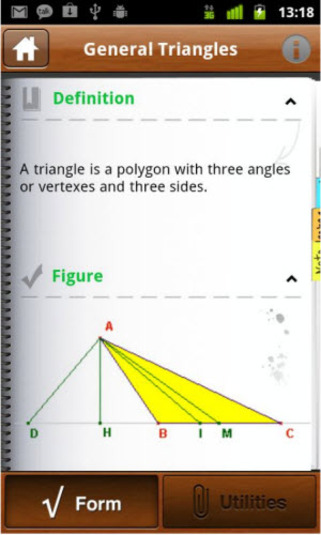# Top 5 Calculator applications for Android and iOS

These days, everyone uses the android and ios mobile phone. In most of the Smartphone, there are pre-installed applications so that the user can use it without installing the new one. Similarly, you can see the calculator that is inbuilt in most of the Smartphone. For some people, it is satisfactory to use the calculator application that is inbuilt rather than installing the new calculator application whereas on the other, some people find it old-fashioned and don’t like the functionality of the application and its look.

###### Well here is top 5 calculator application that you love to use in your android and ios:-

Photomath application

Photomath application is one of the best applications for solving any kind of mathematics problem. Many people are there who don’t like to solve the mathematics problem as they find it very difficult to do so. But with the help of the photomath calculator application, you can solve any kind of mathematical problem in few minutes. You need to simply point the camera at any page or piece of paper with the mathematical equation and you will get the solution of the related problem without any difficulty. You can even use the offers on Amazon to purchase this application.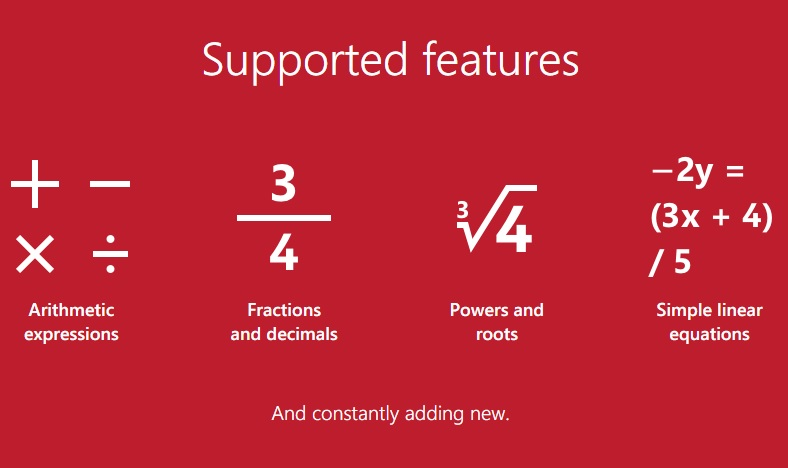Myscript calculator

This is another type of calculator application that will help you to get the solution of your mathematical problem. You can solve the problems related to the square, basic arithmetic, logarithm, trigonometry, and percentages. This application recognizes your handwriting so you can draw any mathematical equation on your phone screen and this application will solve the equation.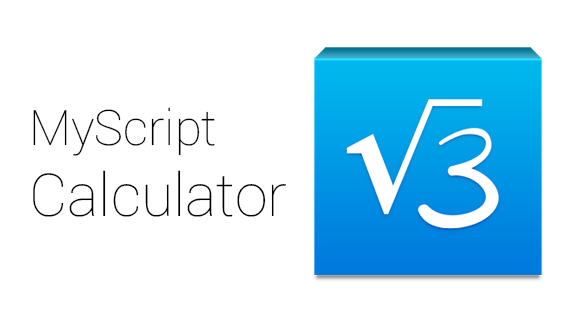Solve4x ios application

This is one of the best free application that you can use for solving the mathematical equation. You can either type the equation on the screen or you can take a snap and it will automatically process the entire equation without any problem. In this way, you can get the appropriate solution to your mathematical problem without any difficulty.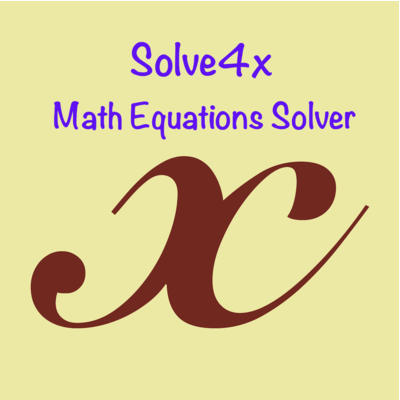Pcalc

There are numbers of scientific calculator available on the ios app store that you can use but one of the best calculator applications that you can use for ios device is the pcalc because it has amazing notification center widget. This means that you don’t have to search the calculator application, you need to just swipe down from the top of your screen and it will get started. In this way, you can find any kind of solution quicker.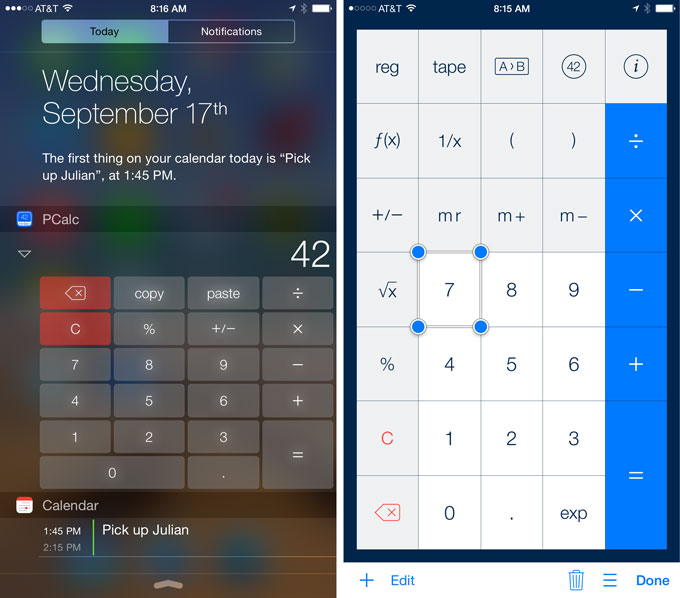Imathematics

You can make the mathematical problems very easy and simple with the help of imathematics application. You need to type the equation of the mathematic problem and you will get the right solution of your problem. Students can also learn the different learning modules that will help them to solve any kind of mathematical complex problem without any difficulty. You can install this application on your Smartphone and can use it for solving any kind of mathematical problem in just a few minutes. In this way, you will be able to get the correct answer.